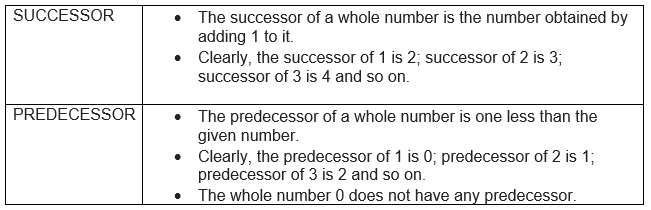# CBSE Notes for Class 6 Maths Chapter 2: Whole Numbers

These are of chapter 2 Whole Numbers class 6 notes. Hope you like these Whole Numbers notes and share the page among your friends.
Table of Content

## Whole Numbers

The numbers 1,2, 3, are called natural numbers or counting numbers.

Let us add one more number i.e., zero (0), to the collection of natural numbers. Now the numbers are 0,1,2, … These numbers are called whole numbers

We can say that whole nos. consist of zero and the natural numbers. Therefore, except zero all the whole nos. are natural numbers.

Facts of Whole numbers

1) The smallest natural number is 1.

2) The number 0 is the first and the smallest whole nos.

3) There are infinitely many or uncountable number of whole-numbers.

4) All natural numbers are whole-numbers.

5) All whole-numbers are not natural numbers. For example, 0 is a whole-number but it is not a natural number.

The first 50 whole nos. are

0, 1, 2, 3, 4, 5, 6, 7, 8, 9, 10, 11, 12, 13,14, 15, 16, 17, 18, 19, 20, 21,

22, 23, 24, 25,26, 27, 28, 29, 30, 31, 32, 33, 34, 35, 36, 37, 38, 39, 40,

41, 42, 43, 44, 45, 46, 47, 48, 49, 50
Some other Important terms to rememberExample
1) Write the successor of
(a) $10701$ (b) $100499$ (c) $5099999$ (d) $5670$
2) Write the predecessor of
(a) $14$ (b) $100000$ (c) $8090$ (d) $4321$
Solution
1)
a) $10702$
b) $100500$
c) $5100000$
d) $5671$
2)
a) $12$
b) $99999$
c) $8089$
d)$4320$

## Properties of Whole Numbers

Topic of Properties of Whole numbers is deleted from this chapter as per rationalized syllabus.

### Closure Property

Closure property on Addition for Whole Number
$0+2 =2$
$1+3=4$
$5+6=11$
So Whole number are closed on Addition
Closure property on Multiplication for Whole Number
$0 \times 2 =0$
$1 \times 4=4$
$5 \times 1 =5$
So Whole number are closed on Multiplication
Closure property on subtraction of Whole number
$5-0 = 5$
$0-5 =?$
$1-3 =?$
$3-1 =2$
So Whole number are not closed on Subtraction
Closure property on Division of Whole number
$\frac {2}{1}= 2$
$\frac {1}{2} =?$
$\frac {0}{2}= 0$
$\frac {2}{0} =?$ ( Division by Zero is undefined)
So Whole Number are not closed on Division
In short
 Closure Property If a and b are any two whole numbers, then a+b, axb are also whole numbers.

### Commutative property

Commutativity property on Addition for Whole Number
$0+2 = 2+0=2$
So Whole number are Commutative on Addition
Commutativity property on Multiplication for Whole Number
$0 \times 2 =0 \; or \; 2 \times 0=0$
So Whole number are Commutative on Multiplication
Commutativity property on subtraction of Whole number
$5-0 = 5$ but $0-5 =?$
So Whole number are not Commutative on Subtraction
Commutativity property on Division of Whole number
$\frac {2}{1}= 2$   but $\frac {1}{2} =?$
So Whole Number are not Commutative on Division
In short
You can add two whole numbers in any order. You can multiply two whole numbers in
any order.
 Commutative property If a and b are any two whole numbers, then $a+b = b+a$ and $a \times b = b \times a$

### Associative property

Associativity property on Addition for Whole Number
$0+(2+3) = (0+2) +3=5$
$1+(2+3) =6= (1+2) +3$
So Whole number are Associative on Addition
Associativity property on Multiplication for Whole Number
$0 \times (2 \times 3) =0$ or $(0 \times 2) \times 3=0$
So Whole number are Associative on Multiplication
Associativity property on subtraction of Whole number
$10-(2-1) = 9$ but $(10-2)-1 =7$
So Whole number are not Associative on Subtraction
Associativity property on Division of Whole number
$16 \div (4 \div 2) = 8$ but $(16 \div 4) \div 2 =2$
So Whole Number are not Associative on Division
So in Short
If a, b and c are any two whole numbers, then (a+b)+c = a+(b+c) and (a×b)×c = a×(b×c).

### Distributive property

If a, b and c are any two whole numbers, then $a(b+c) = a \times b + a \times c$

If a is any whole number, then $a + 0 = a = 0 + a$.
Example
$2+0=2$
$0+3=3$
$5+0=5$

### Multiplicative Identity

If a is any whole number, then $a \times 1 = a = 1 \times a$
Example
$1 \times 1 =1$
$5 \times 1=5$
$6 \times 1=6$

### Multiplication by zero

If a is any whole number, then $a \times 0 = 0 = 0 \times a$.
Example
$1 \times 0 =0$
$5 \times 0=0$
$0 \times 0 =0$

### Division by zero

If a is any whole number, then a ÷ 0 is not defined

### Quiz Time

Question 1 Which of the following is not defined?
A)$10 +0$
B)$10 -0$
C)$10 \times 0$
D)$10 \div 0$
Question 2 Find the value of 6536 91 + 9 6536?
A)588240
B)594776
C)58824
D)653600
Question 3 Which of the following is true
A)Every whole number has predecessor
B)The product of two whole numbers need not to be whole number
C)1 is the identity for multiplication of whole numbers.
D)1 is the identity for addition of whole numbers.
Question 4 Which of the following is not true
A) Whole number are closed on addition
B) Whole number are Commutative on Multiplication
C) Whole number are Commutative on Subtraction
D) Whole number are Commutative on addition
Question 5 The product of a non-zero whole number and its successor is always?
A)even number
B)odd number
C)prime number
D)divisible by 5

• Notes
• Assignments
• NCERT Solutions### Practice Question

Question 1 What is $\frac {1}{2} + \frac {3}{4}$ ?
A)$\frac {5}{4}$
B)$\frac {1}{4}$
C)$1$
D)$\frac {4}{5}$
Question 2 Pinhole camera produces an ?
A)An erect and small image
B)an Inverted and small image
C)An inverted and enlarged image
D)None of the above